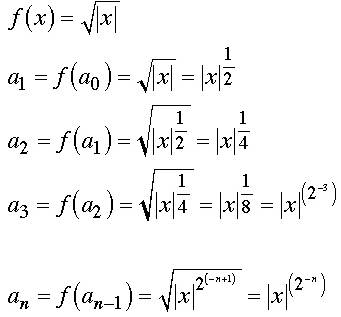# If ao=x, an+1=f(an), n=0,1,2...... find an when        (a)f(x)=sqrt(|x|)                        ans. |x|^(1/2^n)(b) f(x)=1/1-x                          ans. an = x if n=3m, m{0,1,2…}                                                            =1/1-x if n=3m+1                                                            =x-1/x if n=3m+2Plz giv a hint..

10 years ago

If ao=x, an+1=f(an), n=0,1,2...... thenRest i think U should try first!

Plz Approve!